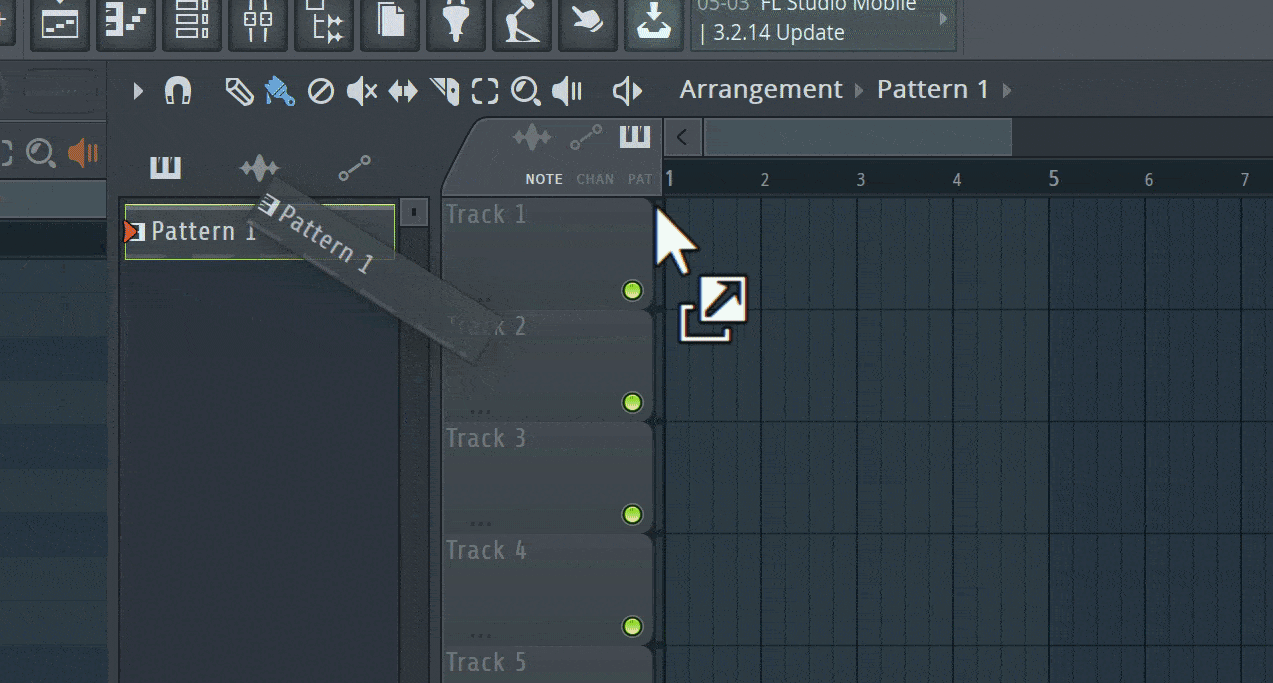# Help with "Drag and drop" animation (Math)

Hello, I need help figuring out the calculation for this drag and drop animation from FL Studio.I would like to recreate this effect but I’m unsure of the calculation to make per frame. I know the basics of this animation but I need someone with more knowlage on animation math.

## Animation properties:

• The box you’re dragging tilts more the faster it is moved across the screen. (DeltaTime + Distance since last frame)
• Spring back/Sway when the box is stationary after being moved.

Thanks for any help!

1 Like
1 Like

I won’t recommend using my library for interpolation or such animations since my resource is expensive in terms of memory and execution and only meant for proper physics simulations.

For this animation I’d recommend checking out Springs. Even tweens would work, but will be janky. You would want to tween the box right below the mouse location.

If you’re using springs for the animation, making constraints between the mouse cursor and the element would be easier. The constraint is what essentially pulls the box towards the mouse cursor. The position for the element being dragged can be calculated each frame as such, here’s some pseudocode:

Assuming Position is the center of the draggable element and the mouse cursor.

``````-- calculating a force to apply to the element
local force = mouse.Position - element.Position
-- 1 is the target magnitude of the constraint
-- you can change 1 to whatever number you want depending on how close or far you want the element to be from the mouse cursor.
local mag = force.Magnitude - 1
-- normalize the force
force = force.Unit
-- let k be some spring stiffness constant. we'll take it as 0.1 for now
-- you can fidget around with this constant to match the results you wish to acheive.
local k = 0.1
-- application of hooke's law
force *= -1 * k * mag

-- finally apply the force to the element.
-- speed is a scalar number
-- dt is the deltaTime
element.Position += speed * force * dt
``````

To tilt the element towards the mouse, this may work till some extent.

``````local dif = mouse.Position - element.Position
element.Rotation = math.deg(math.atan2(dif.Y, dif.X))
``````

You can clamp this rotation if you have any min-max rotation bounds so that the element doesn’t tilt too much.

A more concrete solution to this can be thought of. This is just a gist of what can be done, if I have any more inputs, I’ll be sure to share. This was the first thing that came to mind, if I went wrong somewhere, do correct me.

Hope this helps solve your problem.

2 Likes